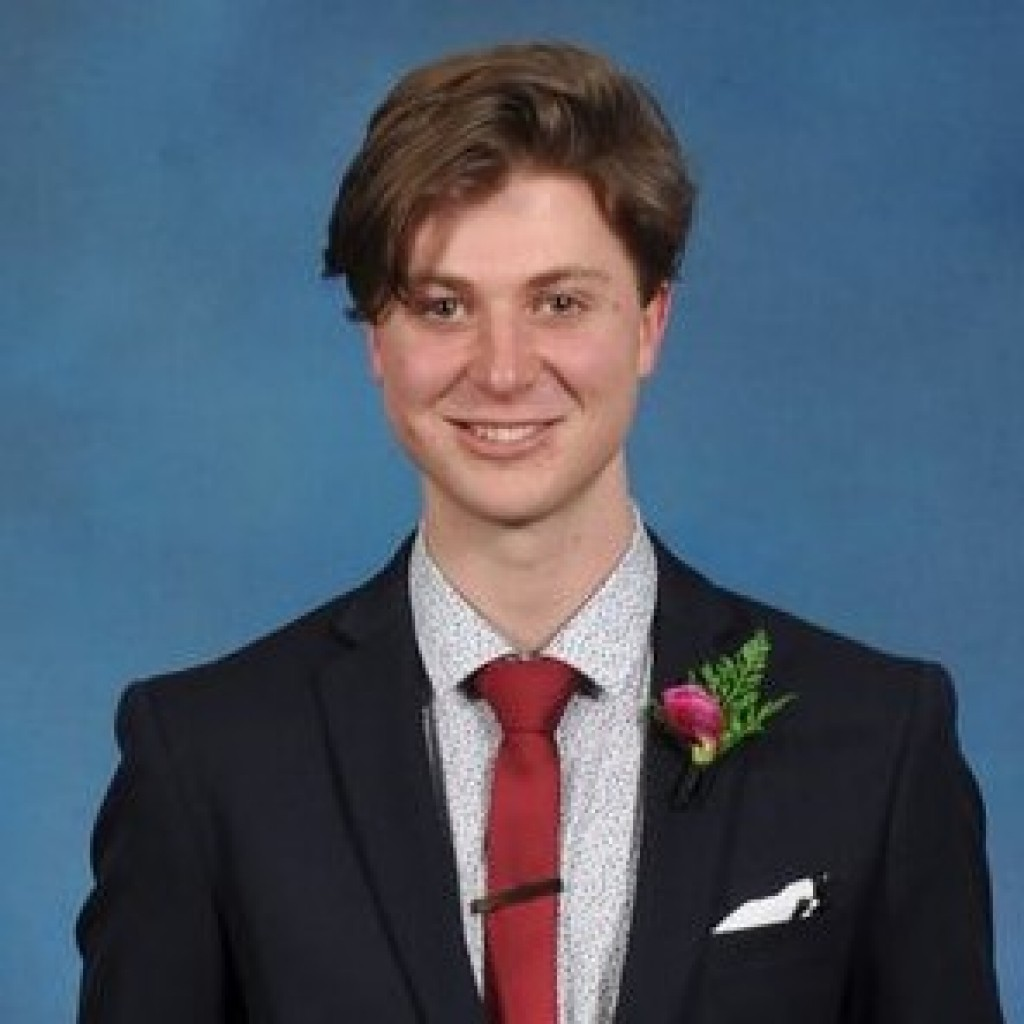Mathematical modelling involves an incredible range of powerful techniques that can be used to describe and predict real-world phenomena. One particular method that is commonly used to model dynamical, or moving, systems is differential equations. These are equations which describe how a variable is changing in relation to another variable, often time.

Melt electrospinning writing is a form of 3D printing that is used to produce microscale objects. It is a relatively new technique and there has not been an abundance of research on it yet. The process and setup are very similar to that of a regular 3D printer, with one major difference. As with other methods, a polymer is inserted and heated so that it melts and is then pushed through a nozzle and deposited on a moving collector plate. However, in melt electrospinning writing the polymer is charged and there is an electric field present between the nozzle and the collector. This creates an electric force on the fibre, which allows for thinner threads to be produced.

The aim of this research was to produce a mathematical model using differential equations to describe melt electrospinning writing. This would allow us to visualise and predict the shape of fibres and hence, ensure that the accuracy of the device is maximised.

Over the course of the AMSI summer research, we built on previous models to derive a system of equations that describe the motion of an electrospun fibre. We were then able to investigate the different variables that could affect the efficiency and accuracy of the process. A range of interesting and potentially useful relationships were determined. Hopefully, with further research and refinement of the model, this work will be able to be utilised to improve the process of melt electrospinning.

One of my key outcomes of this project was a stronger understanding of mathematical models, how they can be formed and how they can be applied. I developed my knowledge of differential equations, as well as learning about 3D printing and melt electrospinning writing.

Jesse TonkinView Jesse Tonkin